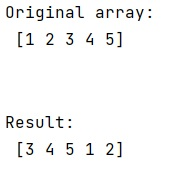# How to wrap around slices in NumPy?

Learn, how to wrap around the slices in Python NumPy?
Submitted by Pranit Sharma, on March 10, 2023

## Wrapping around slices in Python NumPy

Suppose that we are given a numpy array and we need to get a final array as an output where we need an index and its left and right neighbor up to the ith point.

For example, if we are given an array [ 1,2,3,4,5 ] and our target index is 3 and ith point 2 hence, we need 1 neighbor of 4 from left and right. If 4 is the right element, then we need its right neighbor as the first element of the array.

To wrap around slices in NumPy, we can use numpy.take() and set the parameter mode = 'wrap'. This function is used to take elements from an array along an axis. When the axis is not None, this function does the same thing as "fancy" indexing (indexing arrays using arrays); however, it can be easier to use if you need elements along a given axis.

Note: Here, mode specifies how out-of-bounds indices will behave.

Let us understand with the help of an example,

## Python program to wrap around slices in NumPy

```# Import numpy
import numpy as np

# Creating a numpy array
arr = np.array([1,2,3,4,5])

# Display Original array
print("Original array:\n",arr,"\n\n")

# Setting target index
i = 4

# Creating a list of indices
# (the ith points, here we will take 2 neighbours
# from left and right)
ind = range(i-2,i+3)

# Wrapping up the elements
res = arr.take(indices=ind, mode='wrap')

# Display result
print("Result:\n",res)
```

### OutputLanguages: » C » C++ » C++ STL » Java » Data Structure » C#.Net » Android » Kotlin » SQL
Web Technologies: » PHP » Python » JavaScript » CSS » Ajax » Node.js » Web programming/HTML
Solved programs: » C » C++ » DS » Java » C#
Aptitude que. & ans.: » C » C++ » Java » DBMS
Interview que. & ans.: » C » Embedded C » Java » SEO » HR
CS Subjects: » CS Basics » O.S. » Networks » DBMS » Embedded Systems » Cloud Computing
» Machine learning » CS Organizations » Linux » DOS
More: » Articles » Puzzles » News/Updates